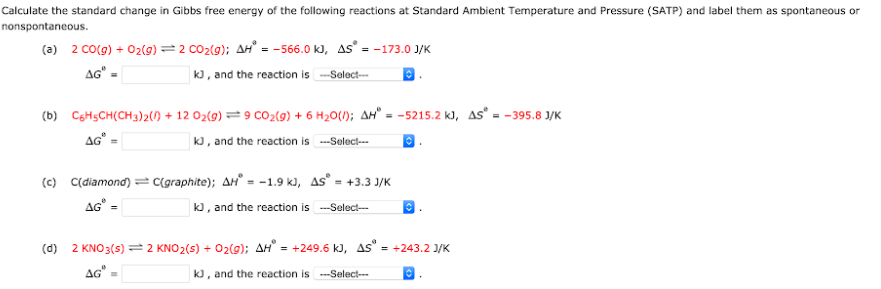# Calculate the standard change in Gibbs free energy of the following reactions at Standard Ambient Temperature and Pressure (SATP) and label them as spontaneous or nonspontaneous. (a) 2 CO(g) + O2(g) <-> 2 CO2(g); ΔH° = -566.0 kJ, ΔS° = -173.0 J/K, ΔG° = ___ kJ, and the reaction is ___ (b) C6H5CH(CCH3)2(l) + 12 O2(g) <-> 9 CO2(g) + 6 H2O (l); ΔH° = -5215.2 kJ, ΔS° = -395.8 J/K ΔG° = ___ kJ, and the reaction is ___. (c) C(diamond <-> C(graphite); ΔH° = -1.9 kJ, ΔS° = +3.3 J/K, ΔG° = ___ kJ, and the reaction is ___. (d) 2 KNO3(s) <-> 2 KNO2(s) + O2(g); ΔH° = +249.6 kJ, ΔS° = +243.2 J/K, ΔG° = ___ kJ, and the reaction is ___.